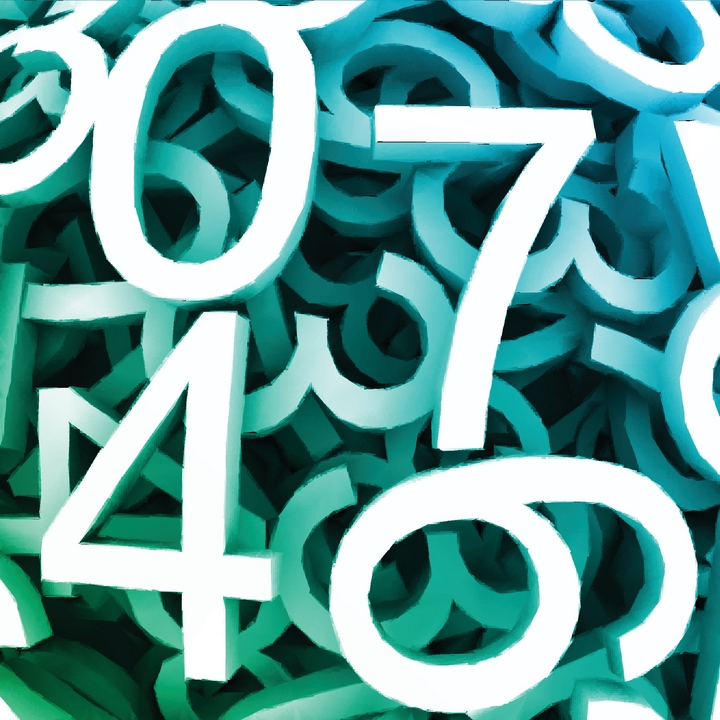# What's the Final Number ?Consider the set

$S= \left \{ 1, \frac {1}{2}, \frac {1}{3}, \frac {1}{4},\cdots, \frac {1}{100} \right \}.$

Choose any two numbers $x$ and $y,$ and replace them with $x+y+ xy.$

For example, if we choose the numbers $\frac{1}{2}$ and $\frac {1}{8}$, we will replace them by $\frac {11}{16}$.

If we keep repeating this process until only $1$ number remains, what is the final number?

×

Problem Loading...

Note Loading...

Set Loading...